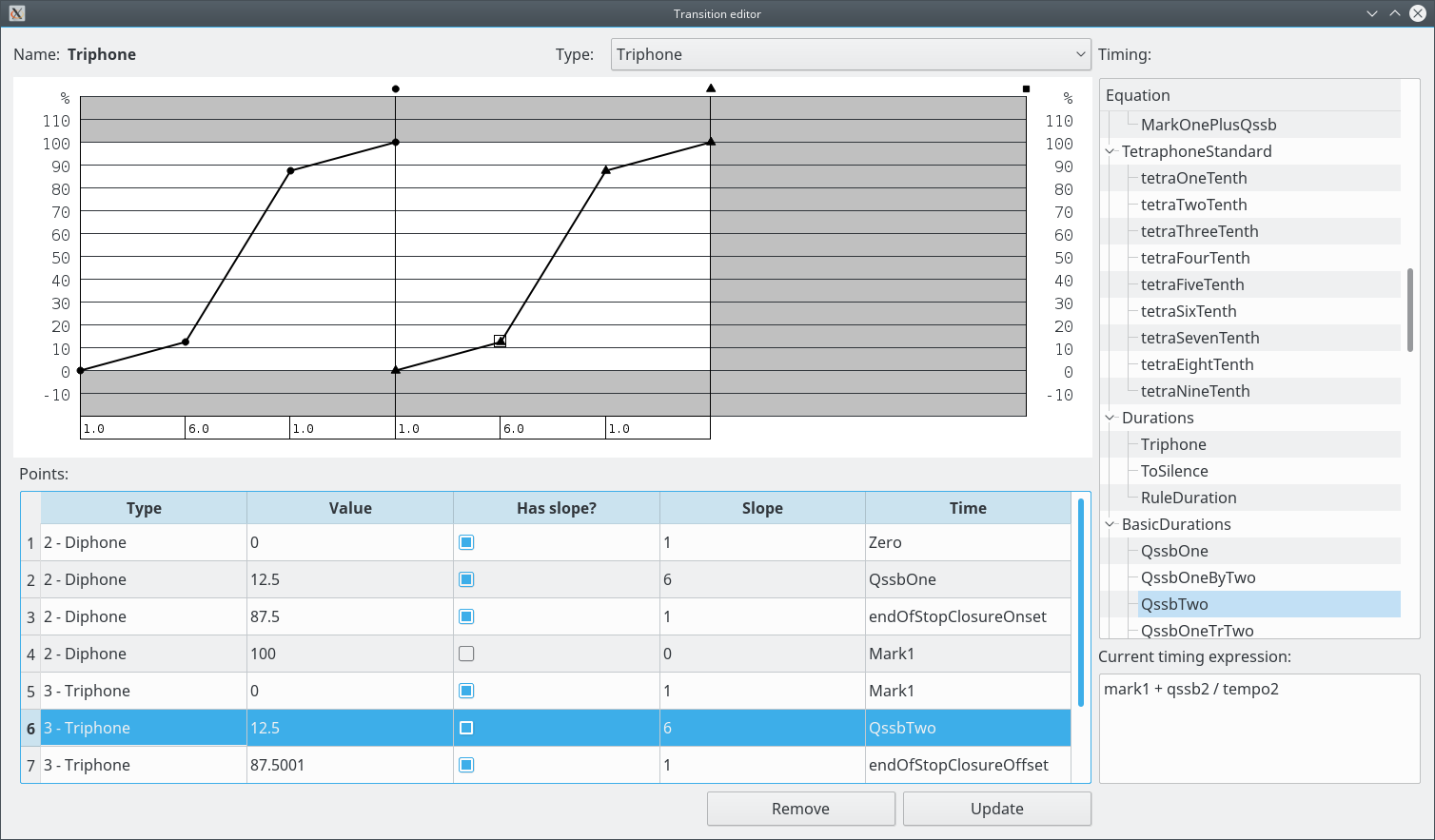# Transition editorTransitions can be diphone (two Postures), triphone (three Postures) or tetraphone (four Postures).

Diphone Transitions have one phase (between Posture 1 and Posture 2), triphone two (between Posture 1 and Posture 2 and between Posture 2 and Posture 3) and tetraphone three (between Posture 1 and Posture 2, between Posture 2 and Posture 3 and between Posture 3 and Posture 4).

The parameter values are linearly interpolated between points.

In each phase, the point values vary from 0 at the start Posture to 100 at the end Posture. The parameter value is calculated by:
`value = start_value + (start_value - end_value) * (point_value / 100)`.

The point values can be defined manually, or by using slopes. In a slope group, the effective slopes of each linear segment are proportional to the defined slope values. This means that the slopes are relative to the other slopes in the group. The slopes are indicated at the bottom of the graph.

In this editor, for the calculation of the point times (using the Equations), the following values are set:
qssa / qssb / transition = 100/3 ms
phase duration = 100 ms
mark1 = 100 ms
mark2 = 200 ms
mark3 = 300 ms
total duration = 100 ms (diphone), 200 ms (triphone), 300 ms (tetraphone)

The points are sorted by type and by time.

The last point of the Transition is ignored (it is a "phantom" point).

The modifications in this window are not executed directly on the main Model. Only when the user clicks on "Update" the changes are sent to the Model.

Operations:

• Select the type of the Transition:
Select the type in the "Type" combobox.
Double click on the graph. The point type is determined by the phase in which the point is created. The point is created with absolute time (the time is not defined by an Equation).
• Select a point:
Click on the graph near the point or select a line in the points table.
• Remove a point:
Select a point and click on "Remove".
• Change the point value:
Select a point, double-click on the "Value" column, enter the value and press ENTER. The value can not be changed if the point is the start of a slope, because in this case the slope calculation is what determines the value of the point.
• Set the point as the start of a slope:
Select a point and check the "Has slope?" column.
• Set the slope:
Select a point, double-click on the "Slope" column, enter the value and press ENTER.
• Set the point time:
Select a point and select an Equation in the Equations tree at the right. It is not possible to clear the time (go back to absolute time).
• Send the changes to the Model:
Click on "Update". Any modifications are lost if this operation is not executed.